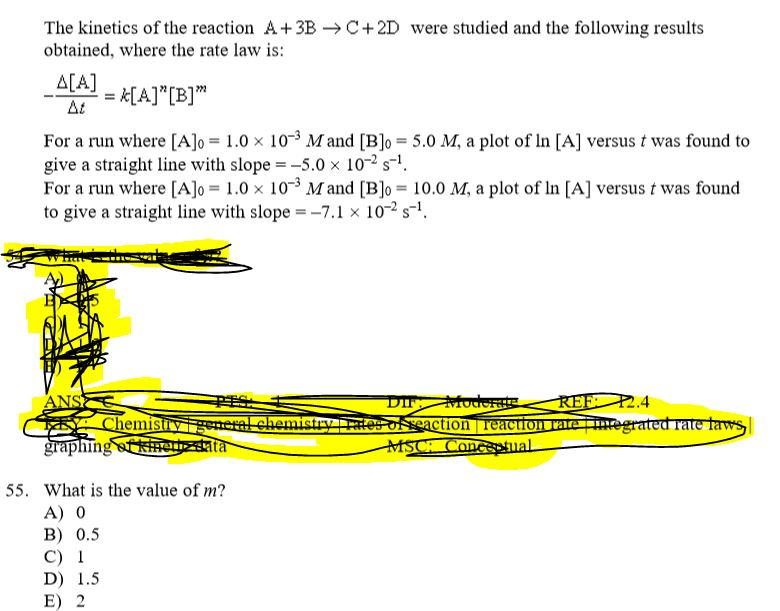# The kinetics of the reaction A + 3B→C + 2D were studied and the following results obtained where the rate law is: -Δ[A]/Δt=k[A]^n[B]^m For a run where [A]o = 1.0×10^-3 and [B]o = 5.0 M, a plot of ln[A] versus t was found to give a straight line with slope =-5.0×10^-2 s^-1. For a run where [A]o = 1.0×10^-3 and [B]o = 10.0 M, a plot of ln[A] versus t was found to give a straight line with a slope = -7.1×10^-2 s^-1. What is the value of m?